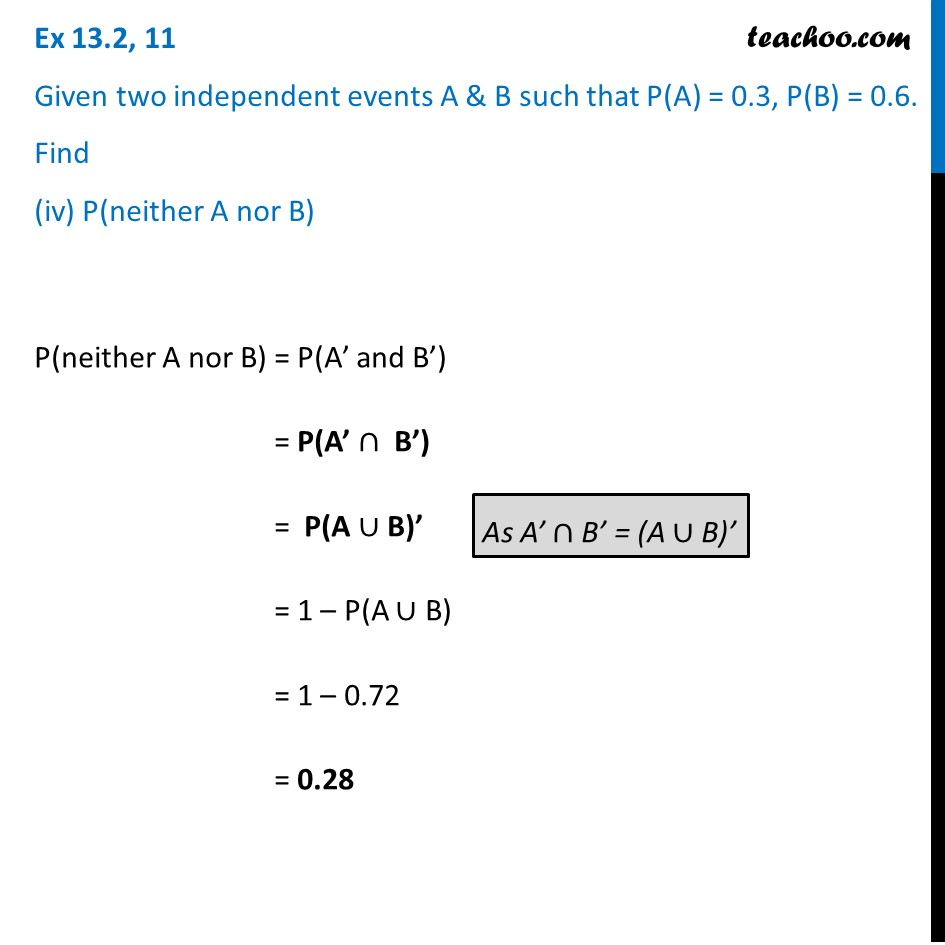Ex 13.2

Chapter 13 Class 12 Probability
Serial order wiseLearn in your speed, with individual attention - Teachoo Maths 1-on-1 Class

### Transcript

Ex 13.2, 11 Given two independent events A & B such that P(A) = 0.3, P(B) = 0.6. Find (iv) P(neither A nor B) P(neither A nor B) = P(A’ and B’) = P(A’ ∩ B’) = P(A ∪ B)’ = 1 – P(A ∪ B) = 1 – 0.72 = 0.28